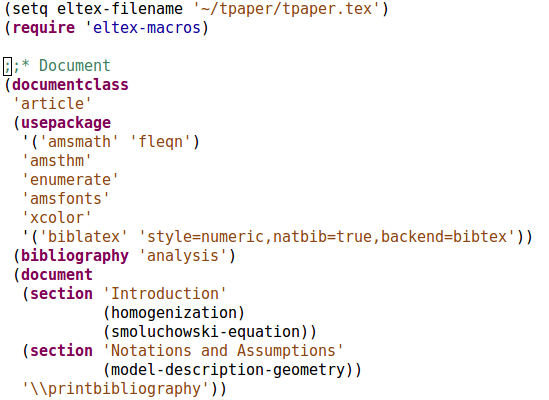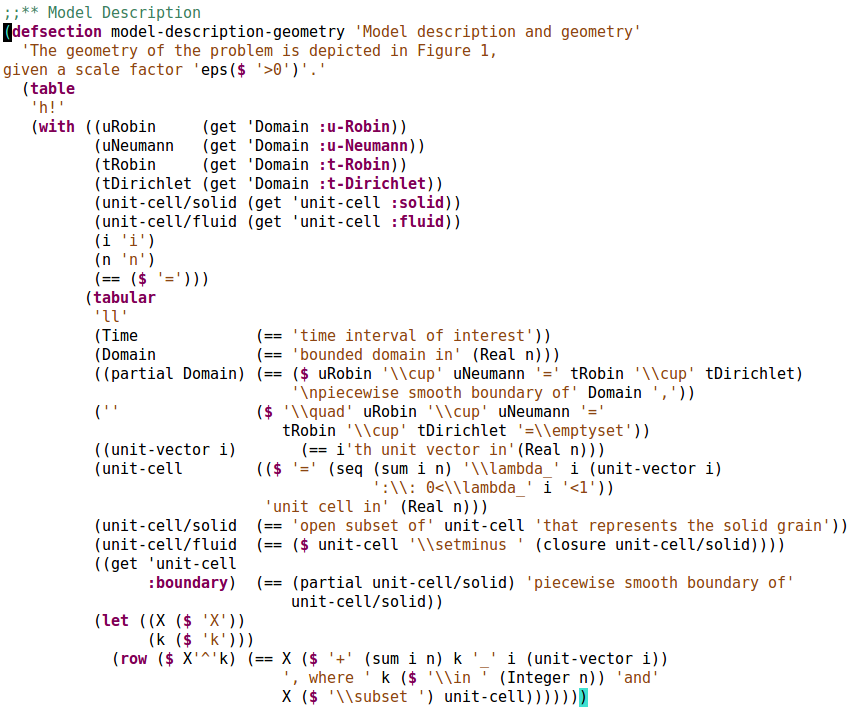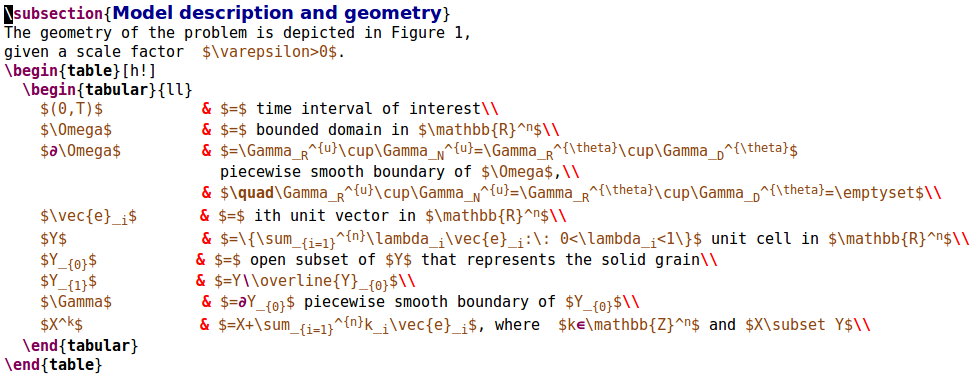ElTeX - generate full LaTeX documents from Emacs Lisp

OK. Please stop laughing. It's a thing now.

Seriously though, it's my little project of writing a major mode for Emacs. I've written many minor modes, but this is my first major one. Granted, it's only a small derivation of emacs-lisp-mode.

How the ElTeX looks like

Here's how a sample document outline looks like:Here, homogenization, smoluchowski-equation, and model-description-geometry are simply Elisp functions that should produce a string on call. They are defined here.

On calling M-x eltex-compile, the corresponding LaTeX document will be written to the file to which eltex-filename points to.

How the corresponding LaTeX looks like

Here's an excerpt of what will be generated:

\documentclass{article}
\usepackage[fleqn]{amsmath}
\usepackage{amsthm}
\usepackage{enumerate}
\usepackage{amsfonts}
\usepackage{xcolor}
\usepackage[style=numeric,natbib=true,backend=bibtex]{biblatex}
\begin{document}

\section{Introduction}

\subsection{Homogenization}

How the original ElTeX looks like in emacs-lisp-mode

(setq eltex-filename "~/tpaper/tpaper.tex")
(require 'eltex-macros)

;;* Document
(documentclass
"article"
(usepackage
'("amsmath" "fleqn")
"amsthm"
"enumerate"
"amsfonts"
"xcolor"
'("biblatex" "style=numeric,natbib=true,backend=bibtex"))
(bibliography "analysis")
(document
(section "Introduction"
(homogenization)
(smoluchowski-equation))
(section "Notations and Assumptions"
(model-description-geometry))
"\\printbibliography"))

Why this?

Well, why not? At one point I was frustrated with LaTeX not allowing me to define mathematically rich entities. I did hack up a few TeX macros for this eventually, but it was very awkward.

So I thought that I could make Emacs Lisp generate simple LaTeX in a similar way that C generates machine code. No more intricate LaTeX macros, only plain LaTeX, generated from (intricate) Elisp.

This is still very much in a toy stage, I don't currently use it for anything serious. Still, in case you're interested in defining derived major modes, you can look at my implementation, it's only 25 lines. The interesting thing about it, if you noticed, is that it replaces all double quoted strings visually with single quoted strings, so that they don't jump out as much.

More examples

Here's how the typesetting of some mathematical logic looks like:And here's the corresponding LaTeX:Note how simple the resulting LaTeX is, considering how many variables were used to generate it. Now, I can update anything in the with Elisp binding, and the corresponding LaTeX will be appropriately regenerated, error-free.